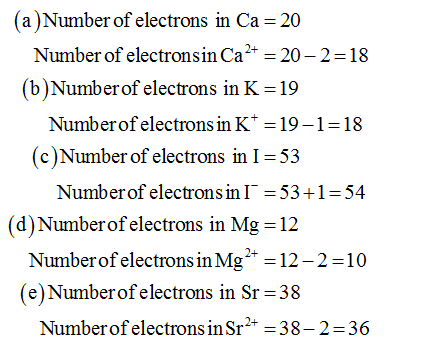# which one of the following ions is isoelectronic with krypton?(a) Ca^2+  (b) K^+   (c) I^-  (d)Mg^2+   (e)Sr^2+

Question
1 views

which one of the following ions is isoelectronic with krypton?

(a) Ca^2+  (b) K^+   (c) I^-  (d)Mg^2+   (e)Sr^2+

check_circle

Step 1

Number of electrons in the given ions is determined as follows,

In a neutral atom, number of electrons will be equal to atomic mass....

### Want to see the full answer?

See Solution

#### Want to see this answer and more?

Solutions are written by subject experts who are available 24/7. Questions are typically answered within 1 hour.*

See Solution
*Response times may vary by subject and question.
Tagged in